# Revision of Second term’ / Re-presentation of real situation an graph and the reason(s).

Welcome to Class !!

In today’s Mathematics class, We will be revising some second term topics such as distance-time Graph and Velocity-Time Graphs. We will also be looking at the Representation of real-life Situations on a Graph. We hope you enjoy the class!

CONTENT

Distance – Time graph

Velocity Time graph

Re-representation of real-life situations on graphs

Choosing scales.

##### Distance – Time Graph

Graphs are used to show the relationship between two quantities. A continuous graph is in the form of a continuous line and shows the relationship between the two quantities.

A distance-time graph shows the distance travelled against the time taken and is used to calculate speeds.

A distance-time graph is also called a Travel graph. In travel graph, the time is usually plotted x-axis and the distance on y-axis.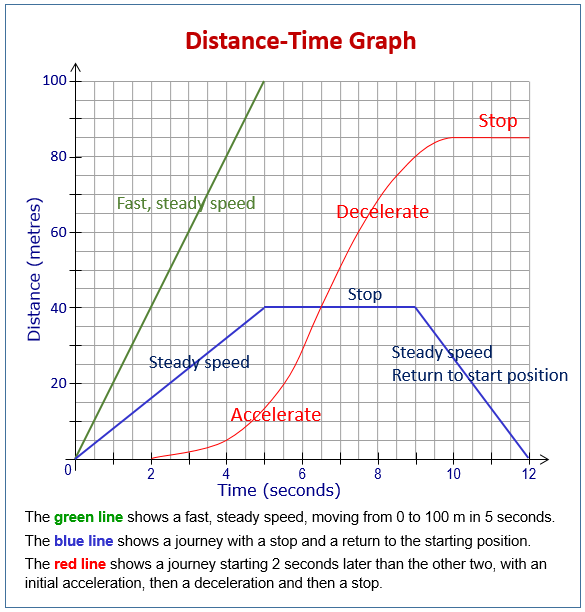Example

The graph below shows a man’s journey from home to another town. Use the graph to find:

• The time taken to travel 75km
• The distance travelled in 3 hours
• The time taken to cover a distance of 175km
• The man’s speed in km/h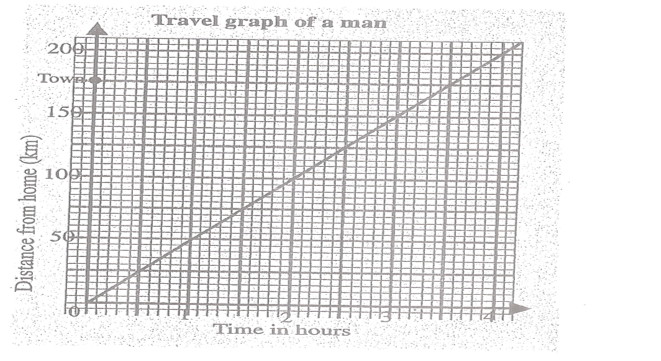Solution with explanation

The horizontal (or x-axis) shows the time in hours.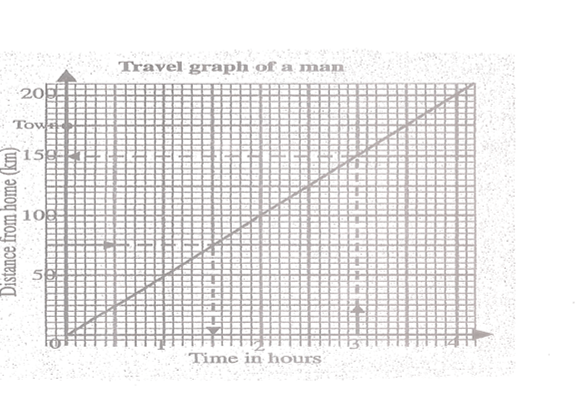2 units on the x-axis = 1 hour

So 1 unit =  hr or 30 mins

The vertical axis (or y-axis) shows the distance in km.

2 units on the y-axis = 50km,

We can use a travel graph to find a distance and time at any point on the graph.

For example:

• The time taken to travel 75km is 1 h 30 mins (see the arrow)
• The distance travelled in 3 hours is 150km (see the arrow)
• It took the man 3 hours 30 mins to cover a distance of 175 km.
• In 3 hrs the man travelled 175km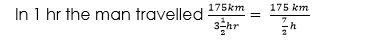= 50km/hr

EVALUATION

1. A girl walks along a road at a speed of 100m per minute
2. Copy and complete the table
 Time(s) 0 1 2 3 4 5 6 Distance(m) 0 100 200

1. Using a scale of 2cm to 1min on the horizontal axis and 2cm to 100m on the vertical axis draw the graph of the information
2. Use the graph to find
3. How far the girl has walked after 4.6mins
4. How long it takes her to walk 380m

Essential Mathematics Chapter 16, pgs. 184-187 AJS Oluwasanmi

Exercise 16.6 Nos 1 & 3 page 201

##### GRAPH OF REAL-LIFE SITUATION

Choosing Scale.

In choosing a scale, choose a big scale while drawing graphs. Refer to the given data to find the upper and lower limit of the scale. Always show the origin if possible on 2cm graph paper use scale of 2cm to 1, 2, 5, 10, 20, 50, 100

GENERAL EVALUATION:

• The labour charges preparing a television set consists of a standing charge of N500 on all bills and an hourly rate of N200 per hour.
1. Make a table showing the total labour charges for jobs which take 1/2h, 1h, 2h, 3h, 4h,
2. Choose a suitable scale and draw a graph of the information
3. Find the total labour charge for a job which takes
• i. 21/2hrs
• ii. 24min
 Time(hr) 1/2 1 2 3 4 Standing 600 700 900 1100 1300

New general mathematics, UBE Edition, chapter 122. Pages 105 – 107

Essential Mathematics by A J S Oluwasanmi, chapter 18, pgs. 187 – 191

WEEKEND ASSIGNMENT

1. Which of the following statements is not true about the equation of the form y = Mx + c?

A. x and y are called the variables.    B. only c is the constant term    C. the graph of this equation is always a straight line     D. the intercept of the graph on the y-axis is c.

The graph below shows the cost of cloth (in naira) and length of cloth (in metre). Use the graph to answer questions 2 to 5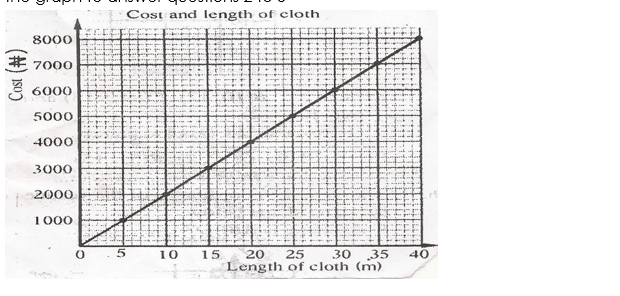1. Find the cost of 12 metres of cloth. N2000     B. N2200    C. N2400     D. N2800
2. Find the cost of 38 metres of cloth N7600     B. N7200      C. N7400     D. N7800
3. How many metres of cloth can be bought for N4400? 20m    B. 25m    C. 24m   D. 21m
4. How many metres of cloth can be bought for N7800? 35m    B. 37m    C. 38m    D. 39m

THEORY

1. Essential mathematics bk. 2 exercise 16.6 number 1 page 201
2. The table below shows the speed of a train at various times
 Time (h) 0 2 4 6 8 10 14 Speed (km/h) 0 15 15 35 35 40 0
• Draw a velocity-time graph for the journey
• Find the acceleration during each stage of the journey
• Find the distance covered by the train in the first 4 hours.

We have come to the end of this class. We do hope you enjoyed the class?

Should you have any further question, feel free to ask in the comment section below and trust us to respond as soon as possible.

In our next class, we will be talking about Angles and Polygons. We are very much eager to meet you there.

How Can We Make ClassNotesNG Better - CLICK to Tell Us💃